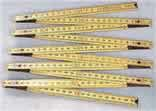Definition of

# Metric SystemA system of measuring based on:

· The meter for length
· The kilogram for mass
· The second for time

Examples:

A kilometer is 1,000 meters
A centimeter is 1/100th (one-hundredth) of a meter
A cubic meter is the volume of a cube whose sides are 1 meter long
A liter is 1/1,000th (one-thousandth) of a cubic meter
A tonne is 1,000 kilograms